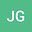loading page

Iterative Splitting Methods for Stochastic Differential Equations: Theory and Application to Coulomb Collisions in Plasma Simulation
•## Abstract

In this paper, we present splitting methods that are based on iterative schemes for stochastic differential equations. The methods are applied to plasma simulations. The motivation arose from solving problems involving Coulomb collisions, which are modeled by nonlinear stochastic differential equations. We apply Langevin equations to model these collisions and we obtain coupled nonlinear stochastic differential equations, which are difficult to solve. We propose stochastic splitting schemes that generalise well-known deterministic splitting schemes. The benefit of decomposing the equations into different parts and solving each part individually is taken into account in the analysis of the new iterative splitting schemes. The increase in the convergence order of the iterative splitting scheme with the number of iteration steps is an important and valuable property. The numerical analysis and applications to various problems involving Coulomb collisions in plasma applications are presented, and show the benefits of the iterative splitting schemes.